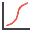RSWebinar: Designing Cost-Effective Ground Improvement Solutions with Settle3 | June 7th, 2023 Register Now

# Cumulative Plot

To plot a Cumulative Distribution after a Probabilistic Analysis:

1. Select Plot Cumulativefrom the toolbar or the Statistics menu.
2. Select a Data Type.
3. When it is relevant, select a Location:
• Single Segment: Select the specific segment you would like to plot from the drop-down menu.
• Multiple Segments: Select the segment(s) you would like to plot. Click Pick Segments and right-click and drag the mouse to select the segments you are interested in. Press ENTER.
• End Wedges: Select the wedge you would like to plot from the drop-down menu.
4. Enter a Number of Intervals. The default is 30, but any value between 1 and 500 can be entered.
5. The display of interval markers may be toggled on or off with the Attach Markers to Distribution checkbox.
6. Select OK.

The Cumulative Distribution will be generated, and the mean, standard deviation, minimum and maximum values for the data plotted, will be listed at the bottom of the plot.

A Cumulative Distribution is, mathematically speaking, the integral of the normalized probability density function. Practically speaking, a point on the cumulative distribution gives us the probability that a random variable will be LESS THAN OR EQUAL TO a specified value.

That is, if (X, Y) is a point on the cumulative distribution S-curve, then Y = the probability that the random variable will be <= X.

The data Sampler allows the user to obtain the coordinates of points on the S-curve.

The same Data Types which can be plotted with Plot Histogram can also be plotted as Cumulative Distributions. See the Data Type topic for details.

## Right-click Options

The following options are available in the right-click menu for Cumulative Distributions: